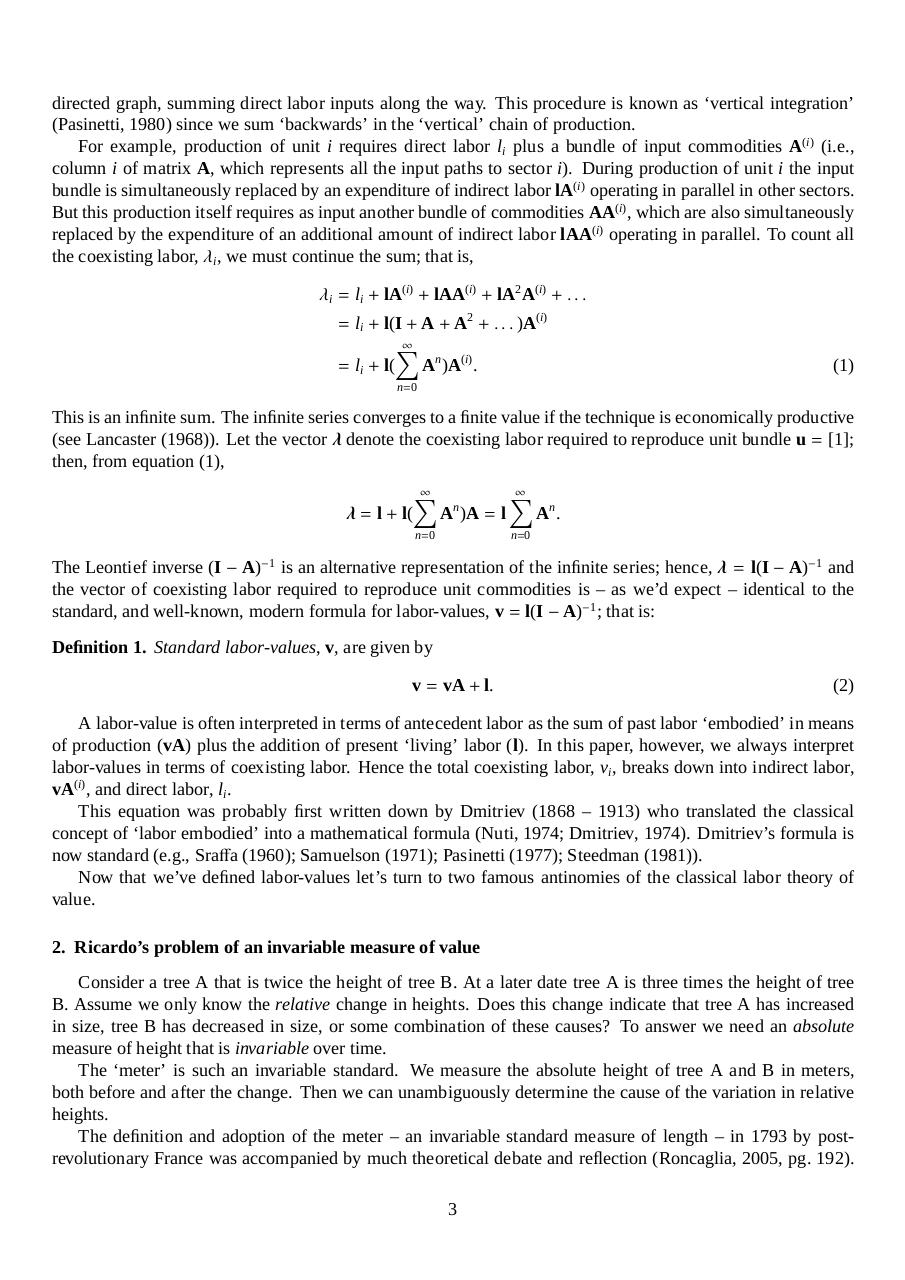# LTV correction on categories transformation problem.pdfPage 1 2 34518

#### Text preview

directed graph, summing direct labor inputs along the way. This procedure is known as ‘vertical integration’
(Pasinetti, 1980) since we sum ‘backwards’ in the ‘vertical’ chain of production.
For example, production of unit i requires direct labor li plus a bundle of input commodities A(i) (i.e.,
column i of matrix A, which represents all the input paths to sector i). During production of unit i the input
bundle is simultaneously replaced by an expenditure of indirect labor lA(i) operating in parallel in other sectors.
But this production itself requires as input another bundle of commodities AA(i) , which are also simultaneously
replaced by the expenditure of an additional amount of indirect labor lAA(i) operating in parallel. To count all
the coexisting labor, λi , we must continue the sum; that is,
λi = li + lA(i) + lAA(i) + lA2 A(i) + . . .
= li + l(I + A + A2 + . . . )A(i)

X
= li + l( An )A(i) .

(1)

n=0

This is an infinite sum. The infinite series converges to a finite value if the technique is economically productive
(see Lancaster (1968)). Let the vector λ denote the coexisting labor required to reproduce unit bundle u = ;
then, from equation (1),

X
X
n
λ = l + l( A )A = l
An .
n=0

n=0

The Leontief inverse (I − A)−1 is an alternative representation of the infinite series; hence, λ = l(I − A)−1 and
the vector of coexisting labor required to reproduce unit commodities is – as we’d expect – identical to the
standard, and well-known, modern formula for labor-values, v = l(I − A)−1 ; that is:
Definition 1. Standard labor-values, v, are given by
v = vA + l.

(2)

A labor-value is often interpreted in terms of antecedent labor as the sum of past labor ‘embodied’ in means
of production (vA) plus the addition of present ‘living’ labor (l). In this paper, however, we always interpret
labor-values in terms of coexisting labor. Hence the total coexisting labor, vi , breaks down into indirect labor,
vA(i) , and direct labor, li .
This equation was probably first written down by Dmitriev (1868 – 1913) who translated the classical
concept of ‘labor embodied’ into a mathematical formula (Nuti, 1974; Dmitriev, 1974). Dmitriev’s formula is
now standard (e.g., Sraffa (1960); Samuelson (1971); Pasinetti (1977); Steedman (1981)).
Now that we’ve defined labor-values let’s turn to two famous antinomies of the classical labor theory of
value.
2. Ricardo’s problem of an invariable measure of value
Consider a tree A that is twice the height of tree B. At a later date tree A is three times the height of tree
B. Assume we only know the relative change in heights. Does this change indicate that tree A has increased
in size, tree B has decreased in size, or some combination of these causes? To answer we need an absolute
measure of height that is invariable over time.
The ‘meter’ is such an invariable standard. We measure the absolute height of tree A and B in meters,
both before and after the change. Then we can unambiguously determine the cause of the variation in relative
heights.
The definition and adoption of the meter – an invariable standard measure of length – in 1793 by postrevolutionary France was accompanied by much theoretical debate and reflection (Roncaglia, 2005, pg. 192).
3